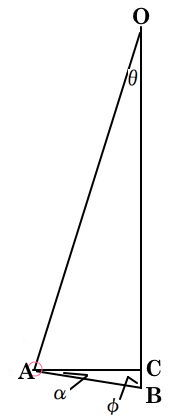Pendulum ```Pendulum period in seconds T ≈ 2π√(L/g) or, rearranging: g ≈ 4π²L/T² L ≈ T²g/4π² L is length of pendulum in meters g is gravitational acceleration (on earth, nominal of 9.8 m/s²) Length for ½ second = 0.062 m Length for 1 second = 0.248 m Length for 2 second = 0.993 m Length for 4 second = 3.97 m Uniform beam pendulum center of oscillation is 2/3 of the length of the uniform beam L from the pivoted end. T ≈ 2π√((2L/3g) Compound pendulum Any swinging rigid body free to rotate about a fixed horizontal axis is called a compound pendulum or physical pendulum. The appropriate equivalent length L for calculating the period of any such pendulum is the distance from the pivot to the center of oscillation. This point is located under the center of mass at a distance from the pivot traditionally called the radius of oscillation, which depends on the mass distribution of the pendulum. If most of the mass is concentrated in a relatively small bob compared to the pendulum length, the center of oscillation is close to the center of mass. The radius of oscillation or equivalent length L of any physical pendulum can be shown to be: L = I/mR where I is the moment of inertia of the pendulum about the pivot point, m is the mass of the pendulum, and R is the distance between the pivot point and the center of mass. The period of a compound pendulum is given by T = 2π√(I/mgR) for a uniform rod pivoted at one end, I = mL²/3 T = 2π√(2L/3g) Speed of bob at lowest pointO is the pivot point of the pendulum. A is the release point B is the point of lowest travel θ is the deflection angle at release φ is angle ABO α is angle BAC L = OA = OB = length of string OC = OA cos θ h = BC = OA – OC = OA – OA cos θ = OA (1 – cos θ) Now use KE = PE to get the speed ½mV² = mgh V = √(2gh) Pendulum period in sec for larger angle, θ in radians T ≈ [2π√(L/G)] [1 + (1/16)θ² + (11/3071)θ⁴ + (173/737280)θ⁶ + (22931/1321205760)θ⁸ + ... ] T ≈ [2π√(L/G)] [1 + (1/2)²(sin²(θ/2) + ((1·3)/(2·4))²(sin⁴(θ/2) + ((1·3·5)/(2·4·6))²(sin⁶(θ/2) + .. ] Conical Pendulum period T = 2π√(r/(gtanθ)) r is radius of the rotation θ is angle of bob with vertical v = 2πr/T v is velocity Change in simple pendulum period due to length change dT/dL = (1/2)2π√(1/g)√1/L) = π√(1/Lg) dT = (π/√(gL))dL for a 1% change in length, what is time change for 1 day, 86400 seconds? dL/L = 0.01 (ie, 1%) dL = 0.01L dT = π/√(gL) (0.01)L dT = (0.01)(π/√g) L/√L dT = (0.01)(π/√g)√L dT = (0.01)(π√(L/g)) dT = (0.01)(1/2)(2π√(L/g)) dT = (0.005)T dT = (0.005)(86400) = 432 seconds Conical Pendulum where the bob moves in a circle. T = tension in string of length L m = mass of bob θ = angle of string with vertical t = 2π√(Lcosθ/g) Compound Pendulum where the rod is not massless or the bob is extended. T ≈ 2π√(I/mgL) g is gravitational acceleration = 9.8 m/s² I is moment of inertia around the pivot in kg·m² m is the mass of the pendulum in kg L is the distance from the pivot to the center of mass of the pendulum in meters Moment of inertia, I, in kg·m² I = cMR² M is mass (kg), R is radius (meters) c = 1 for a ring or cylinder c = 2/5 solid sphere c = ½ solid cylinder or disk around its center c = 1/12 rod around its center, R = length ``` Home Area, Volume Atomic Mass Black Body Radiation Boolean Algebra Calculus Capacitor Center of Mass Carnot Cycle Charge Chemistry   Elements   Reactions Circuits Complex numbers Constants Curves, lines deciBell Density Electronics Elements Flow in fluids Fourier's Law Gases Gravitation Greek Alphabet Horizon Distance Interest Magnetics Math   Trig Math, complex Maxwell's Eq's Motion Newton's Laws Octal/Hex Codes Orbital Mechanics Particles Parts, Analog IC   Digital IC   Discrete Pendulum Planets Pressure Prime Numbers Questions Radiation Refraction Relativistic Motion Resistance, Resistivity Rotation Series SI (metric) prefixes Skin Effect Specific Heat Springs Stellar magnitude Thermal Thermal Conductivity Thermal Expansion Thermodynamics Trigonometry Units, Conversions Vectors Volume, Area Water Wave Motion Wire, Cu   Al   metric Young's Modulus